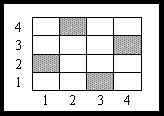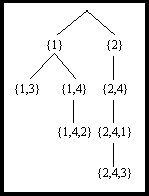# 5.8 Backtracking.

The backtracking method is based on the systematically inquisition of the possible solutions where through the procedure ,set of possible solutions are rejected before even examined so their number is getting a lot smaller.

An important requirement which must be fulfilled is that there must be the proper hierarchy in the systematically produce of solutions so that sets of solutions that do not fulfill a certain requirement are rejected before the solutions are produced.For this reason the examination and produce of the solutions follows a model of non-cycle graph for which in this case we will consider as a tree.The root of the tree represents the set of all the solutions.Nodes in lower levels represent even smaller sets of solutions ,based on their properties.Obviously,leaves will be isolated solutions.It is easily understood that the tree (or any other graph) is produced during the examination of the solutions so that no rejected solutions are produced.When a node is rejected,the whole sub-tree is rejected,and we backtrack to the ancestor of the node so that more children are produced and examined.Because this method is expected to produce subsets of solutions which are difficult to process,the method itself is not very popular.

THE QUEENS PROBLEM

We consider a grid of squares,dimensioned nXn,partly equivalent to a chessboard containing n2 places.A queen placed in any of the n2 squares controls all the squares that are on its row,its column and the 450 diagonals.The problem asked,is how to put n queens on the chessboard,so that the square of every queen is not controlled by any other queen.Obviously for n=2 there is no problem to the solution,while for n=4 a valid solution is given by the drawing below.A possible position on the grid is set by the pair of pointers (i,j) where 1<i,j<n , and i stands for the number of column and j stands for the number of row.Up to this point,for the same i there are n valid values for j.For a candidate solution though,only one queen can be on each column,that is only one value j=V(i).Therefor the solutions are represented with the n values of the matrix V=[V(1),...V(n)].All the solutions for which V(i)=V(j) are rejected because 2 queens can not be on the same row.Now the solutions are the permutes of n pointers,which is n! ,still a forbiddingly big number.Out of all these solution the correct one is the one which satisfies the last requirement:2 queens will not belong in the same diagonal,which is:

V(j)-V(i)<>±(i-j) for i<>j. (5.8-1)

A backtracking algorithm or this problem constructs the permutes [V(1),....V(n)] of the {1,...,n} pointers,and examines them as to the property (5.8-1).For example there are (n-2)! permutes in the shape of [3,4....].These will not be produced and examined if the systematically construction of them has already ordered them in a sub-tree with root [3,4] which will be rejected by the 5.8-1 condition,and will also reject all the (n-2)! permutes.On the contrary ,the same way of producing-examining will go even further to the examination of more permutes in the shape of p={1,4,2,...} since, so far the condition is satisfied.The next node to be inserted that is j:=V(4) must also satisfies these:j-1<>3,j-4<>2,j-4<>-2,j-2<>1,j-2<>-1.All the j pointers satisfying these requirements produce the following permutes:[1,4,2,j,...] which connect to the tree as children of p.Meanwhile large sets of permutes such as [1,4,2,6,...] have already been rejected.

A typical declaration of this algorithm:The root of all solutions,has as children n nodes ,...,[n],where [j] represents all the permutes starting with j(and whose number is (n-1)! for every j).Inductive if a node includes the k nodes {j1,...jk} we attempt to increase it with another node { j1,...,jk,jk+1} so that the condition (5.8-1) is fulfilled.

For n=4 this method produces the indirect graph of the following picture,and does not produce the 4!=24 leaves of all the candidate solutions.Solution to the queens problem using Backtracking (n=4)

HTML PAGE DIRECTOR :Papaioannou Panagiotis